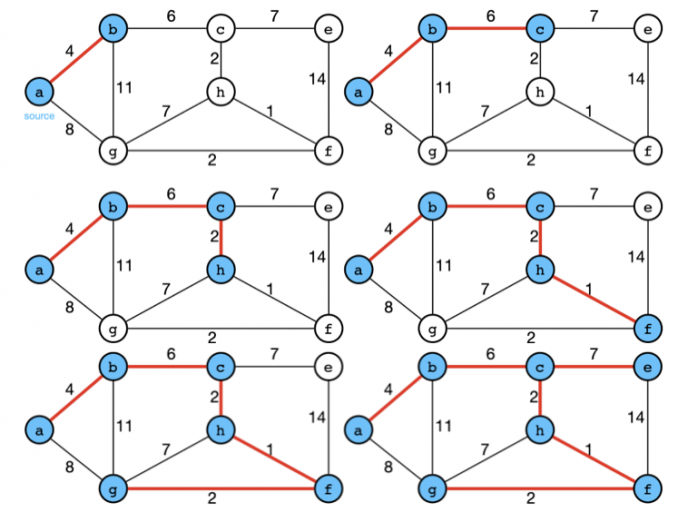# Minimum Spanning Tree — PrimThe idea behind Prim's algorithm is simple, a spanning tree means all vertices must be connected. So the two disjoint subsets (discussed above) of vertices must be connected to make a Spanning Tree. And they must be connected with the minimum weight edge to make it a Minimum Spanning Tree.

This post will introduce one of the algorithms to find an MST: Prim. Logics, time/space complexities, and implementations will be provided. Feat. Leetcode challenge 1584. Min Cost to Connect All Points.

## Logic

Prim’s logic is quite similar to Dijkstra’s algorithm, which also finds the lightest edge greedily. It starts with an arbitrary vertex `v` and ends up with a set of edges `A` spanning all the vertices. At each step, it would look for the edge that connects `A` to an isolated vertex in the graph. The pseudo-code is shown as below.

``````MST-PRIM(G,w,r)
'''
G: the connected graph
w: weights
r: the root vertex
Q: a min-priority queue based on a key field
key: for each vertex v, key[v] is the minimum weight \
of any edge connecting to a vertex in the MST
π: for each vertex v, π[v] names the parent of v in the MST
'''
for each u in V[G]
key[u] = inf
π[u] = NIL
key[r] = 0
Q = V[G]
while Q:
u = EXTRACT_MIN(Q)
if v in Q and w(u,v)<key[v]
π[v] = u
key[v] = w(u,v)``````

Here’s an illustration for Prim:## Complexities

There are two ways of implementations for line 17.

• using adjacency matrix, so that EXTRACT_MIN takes O(V);
• using the binary heap, so that EXTRACT_MIN takes O(logV).

• Initialization takes O(V)(Line 11–15).
• Line 16 would be executed for O(V) time, so EXTRACT_MIN takes O(VV) in total.
• As the while-loop in line 16 will be executed for each vertex and the for-loop in line 18 will be executed for each edge of the vertex, therefore the for-loop in line 18 will be executed for O(E) times.

Therefore, the total time complexity would be O(V+V^_2+_E)=O(V^_2). The space complexity would be _O(V+E).

### Binary heap

• Initialization takes O(V)(Line 11–15).
• Line 16 would be executed for O(V) time, so EXTRACT_MIN takes O(VlogV) in total.
• As the while-loop in line 16 will be executed for each vertex and the for-loop in line 18 will be executed for each edge of the vertex, therefore the for-loop in line 18 will be executed for O(E) times.
• Line 21 indicates an update operation on the heap, thus taking logV.

Therefore, the total time complexity would be O(V+VlogV+ElogV)=O(ElogV). The space complexity would be O(V+E).

## Coding 101: Programming Language Building Blocks

This article will introduce the concepts and topics common to all programming languages, that beginners and experts must know!

## 13 Free/Low-Cost Sites to Supercharge Your Programming Self-Education

Although we still talk about programming as a standalone career, the dominance of technology in our lives makes it clear that coding is much more than a career path. In my opinion, computer science is more than a college major or a high-paid job; it’s a skill, essential for thriving in a modern-day economy. Whether you work in healthcare, marketing, business, or other fields, you will see more coding and have to deal with a growing number of technologies throughout your entire life.

## What Is Code Golfing And Biggest Such Tournaments

Code golf is a type of recreational computer programming competition in which participants strive to achieve the shortest possible source code that implements a certain algorithm. Playing code golf is known as "golf scripting". Code golf challenges and tournaments may also be named with the ... Some code golf questions, such as those posed on general programming ...

## How to Structure Your Programming Code

I remember my first fumble with basic on my ZX Spectrum computer back in the 1980s, ploughing through pages of basic commands and example code without any real idea of how I could write programs myself

## A Guide to Important Graph Algorithms for Competitive Programming

And how you can use DFS and BFS. If you’ve read an Introduction to Competitive Programming, then you’re probably familiar with why Competitive Programming is important.